## Linear Equations: Solutions Using Matrices with Three Variables

Solving a system of equations by using matrices is merely an organized manner of using the elimination method.

##### Example 1

Solve this system of equations by using matrices.The goal is to arrive at a matrix of the following form.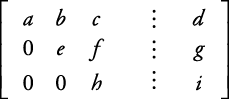To do this, you use row multiplications, row additions, or row switching, as shown in the following.

Put the equation in matrix form.Eliminate the x‐coefficient below row 1.Eliminate the y‐coefficient below row 5.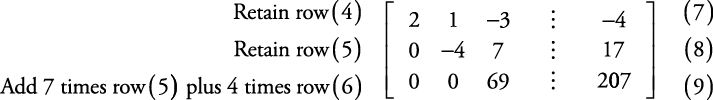Reinserting the variables, this system is nowEquation (9) now can be solved for z. That result is substituted into equation (8), which is then solved for y. The values for z and y then are substituted into equation (7), which then is solved for x.The check is left to you. The solution is x = 2, y = 1, z = 3.

##### Example 2

Solve the following system of equations, using matrices.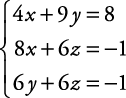Put the equations in matrix form.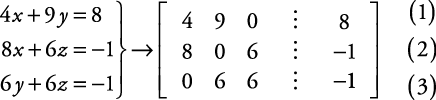Eliminate the x‐coefficient below row 1.Eliminate the y‐coefficient below row 5.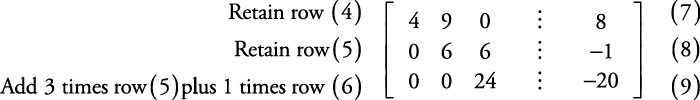Reinserting the variables, the system is now: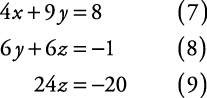Equation (9) can be solved for z.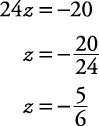Substituteinto equation (8) and solve for y.Substitute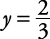into equation (7) and solve for x.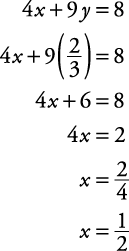The check of the solution is left to you. The solution is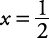,,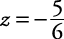.

Back to Top
A18ACD436D5A3997E3DA2573E3FD792A